### Chemical and Process Engineering Resources## Correlations for Convective Heat Transfer

Nov 08 2010 01:20 PM | bspang in Heat TransferIn many cases it's convenient to have simple equations for estimation of heat transfer coefficients. Below is a collection of recommended correlations for single-phase convective flow in different geometries as well as a few equations for heat transfer processes with change of phase. Note that all equations are for mean Nusselt numbers and mean heat transfer coefficients.

Â

Section 1: Forced Convection Flow Inside a Circular Tube

Â

All properties at fluid bulk mean temperature (arithmetic mean of inlet and outlet temperature).

Nusselt numbers Nu0 from sections 1-1 to 1-3 have to be corrected for temperature-dependent fluid properties according to section 1-4.Eq. (1)

Â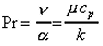Eq. (2)

ÂEq. (3)

Â

1-1 Thermally Developing, Hydrodynamically Developed Laminar Flow (Re<2300)

Constant wall temperature:(Hausen) Eq. (4)

Â

Constant wall heat flux: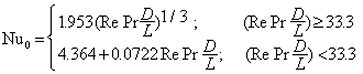(Shah) Eq. (5)

Â

1-2 Simultaneously Developing Laminar Flow (Re<2300)

Constant wall temperature: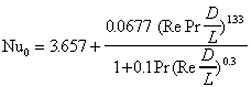(Stephan) Eq. (6)

Â

Constant wall heat flux: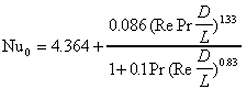Eq. (7)

which is valid over the range 0.7 < Pr < 7 or if Re Pr D/L < 33 also for Pr > 7.

1-3 Fully Developed Turbulent and Transition Flow (Re>2300)

Constant wall heat flux:(Petukhov, Gnielinski) Eq. (8) where: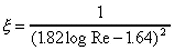Constant wall temperature:

For fluids with Pr > 0.7 correlation for constant wall heat flux can be used with negligible error.

1-4 Effects of Property Variation with Temperature

Liquids, laminar and turbulent flow:Eq. (9)

Subscript w: at wall temperature, without subscript: at mean fluid temperature.

Gases, laminar flow:

 Nu = Nu0 Eq. (10)

Gases, turbulent flow:Eq. (11)

Temperatures in Kelvin.

Section 2: Forced Convection Flow Inside Concentric Annular Ducts, Turbulent (Re > 2300)Dh = Do - Di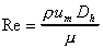All properties at fluid bulk mean temperature (arithmetic mean of inlet and outlet temperature).Â

Heat transfer at the inner wall, outer wall insulated: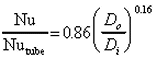(Petukhov and Roizen) Eq. (12)

Heat transfer at the outer wall, inner wall insulated: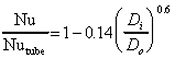(Petukhov and Roizen) Eq. (13)

Heat transfer at both walls, same wall temperatures: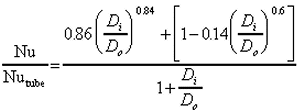(Stephan) Eq. (14)

Section 3: Forced Convection Flow Inside Non-Circular Ducts, Turbulent (Re > 2300)

Equations for circular tube with hydraulic diameter: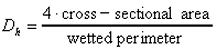Eq. (15)

ÂEq. (16)

ÂEq. (17)

### Heat Transfer Articles

•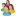Industrial Forum

•Student Forum

•Refining Forum

•Simulation Forum

•Relief Devices Forum

•Tank Venting Forum

•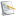Separation Technology

•Calculations and Tips

•Fluid Flow

•Heat Transfer

•Maintenance and Repair

•Utilities

•Safety and Pressure Relief

•Bulk Solids

•Process and Reactions

•Energy

•Other Topics

•For Students

•Physical Properties

•Archived Articles